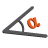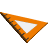You are here: Start » AVL.NET » Geometry 2D Angle Metrics

# Geometry 2D Angle Metrics

Icon Name DescriptionAngleBetweenDirections Measures the rotation angle from first to second direction.AngleBetweenLines Measures the smaller and the larger angle between two lines.AngleBetweenSegments Measures the angle between two segments with one of four possible metrics.AngleBetweenThreePoints Measures the angle defined by three consecutive points.AngleBetweenVectors Measures the angle between two vectors.NormalizeAngle Normalizes an angle to the range <x, x+180) or <x, x+360).